Question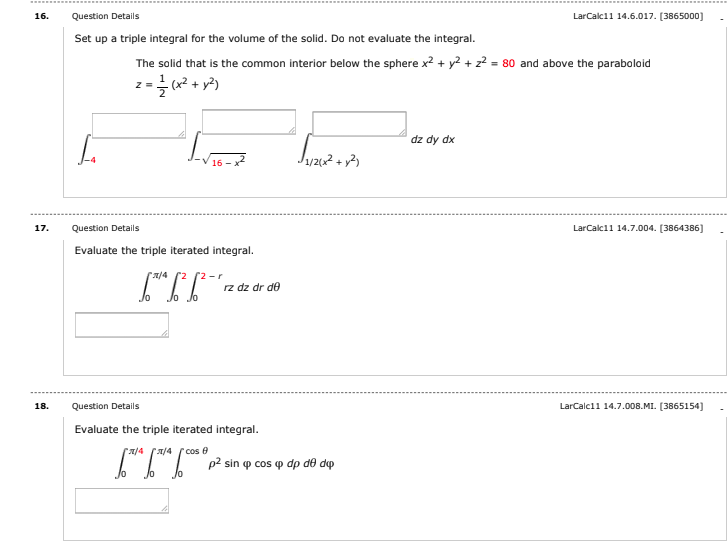We need at least 10 more requests to produce the answer.

0 / 10 have requested this problem solution

The more requests, the faster the answer.

All students who have requested the answer will be notified once they are available.

#### Earn Coins

Coins can be redeemed for fabulous gifts.

Similar Homework Help Questions
• ### Question Use cylindrical coordinates to set up the triple integral needed to find the volume of...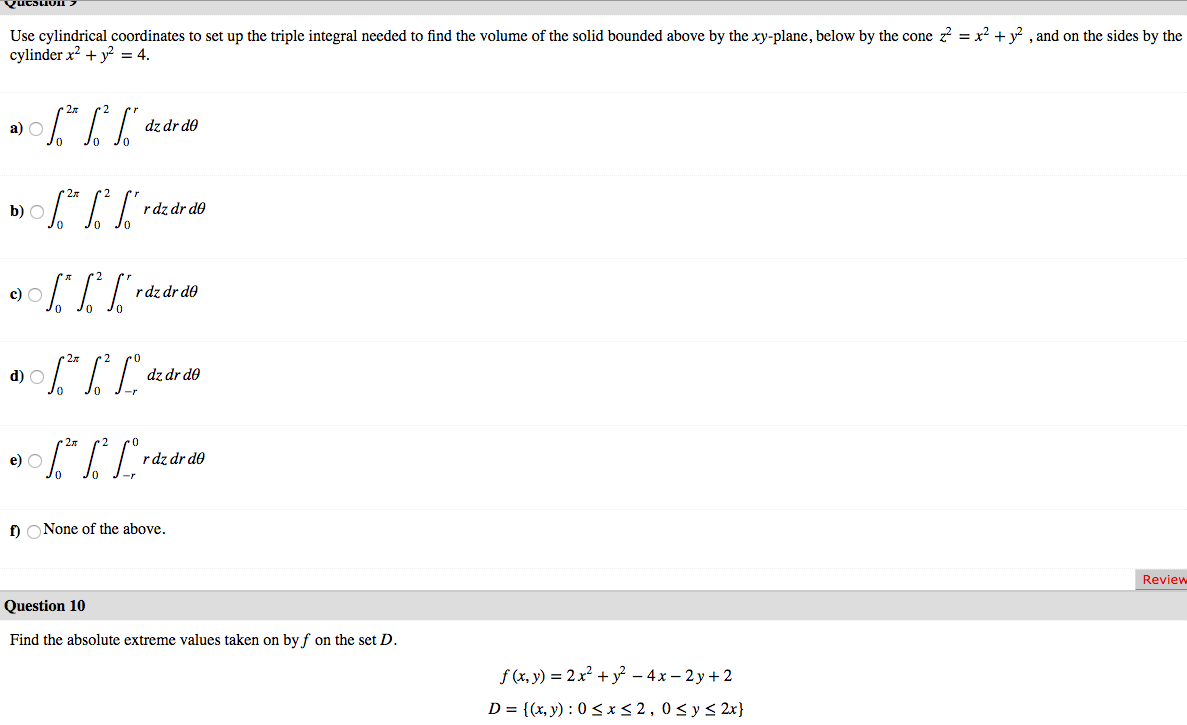Question Use cylindrical coordinates to set up the triple integral needed to find the volume of the solid bounded above by the xy-plane, below by the cone z = x2 + y2 , and on the sides by the cylinder x2 + y2 = 4. a) 06.* %* ["dz dr do b) \$* * S*rde de do JO 0% ] raz dr do a) \$** [Lºdz dr do 0906.*|*Lºrdz dr do 2 po dz dr do Jo J- O J-...

• ### 3. Consider the triple integral 2z sin(x2 + y2 +22 - 2x) dy da dz. Set...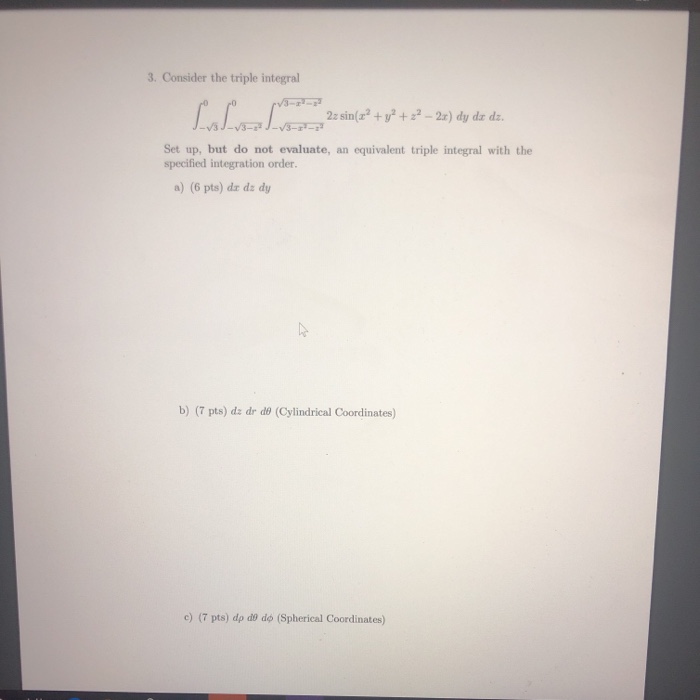3. Consider the triple integral 2z sin(x2 + y2 +22 - 2x) dy da dz. Set up, but do not evaluate, an equivalent triple integral with the specified integration order. a) (6 pts) da dz dy b) (7 pts) dz dr de (Cylindrical Coordinates) c) (7 pts) dp do do (Spherical Coordinates)

• ### Use a triple integral to find the volume of the solid region inside the sphere ?2+?2+?2=6...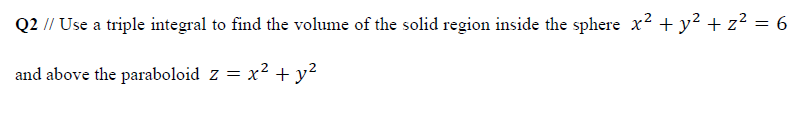Use a triple integral to find the volume of the solid region inside the sphere ?2+?2+?2=6 and above the paraboloid ?=?2+?2 This question is in Thomas Calculus 14th edition chapter 15. Q2 // Use a triple integral to find the volume of the solid region inside the sphere x2 + y2 + z2 = 6 and above the paraboloid z = x2 + y2

• ### 2 147 a. Evaluate the triple integral (convert to oylindrical)12I, J xz dz dx dy b. Find the mome...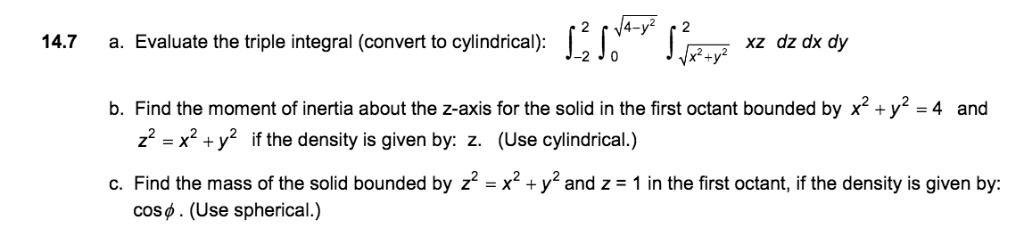2 147 a. Evaluate the triple integral (convert to oylindrical)12I, J xz dz dx dy b. Find the moment of inertia about the z-axis for the solid in the first octant bounded by x2+y2 -4 and z2-x2 + y2 if the density is given by: z. (Use cylindrical.) c. Find the mass of the solid bounded by z2 -x2 +y2 and z 1 in the first octant, if the density is given by: cos. (Use spherical.) 2 147 a. Evaluate...

• ### 8. Clearly construct a triple integral of the form dz dy dr to find the volume...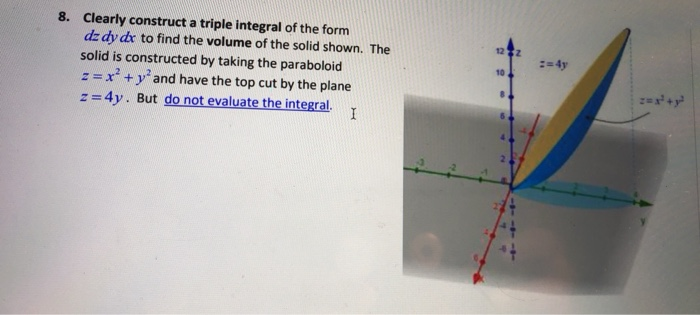8. Clearly construct a triple integral of the form dz dy dr to find the volume of the solid shown. The solid is constructed by taking the paraboloid :=x2 + y and have the top cut by the plane z=4y. But do not evaluate the integral. 1 10 8 ry

• ### Set up, but do not evaluate, a triple integral in cylindrical coordinates that gives the volume...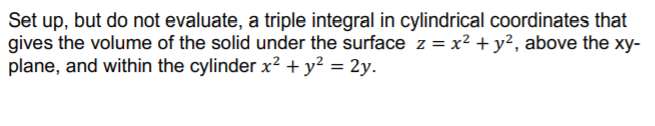Set up, but do not evaluate, a triple integral in cylindrical coordinates that gives the volume of the solid under the surface z = x2 + y2, above the xy- plane, and within the cylinder x2 + y2 = 2y.

• ### 16. o integrad [**** The triple da dy dz describes the solid pictured at right. Rewrite...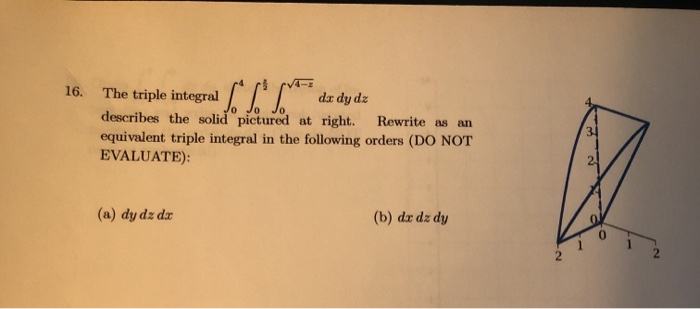16. o integrad [**** The triple da dy dz describes the solid pictured at right. Rewrite as an equivalent triple integral in the following orders (DO NOT EVALUATE): 31 (a) dy dz dx (b) du dz dy 2. 16-2 21. Given dy da, 16- (a) Sketch the region of integration and write an equivalent iterated integral in the order dx dy. (You do not need to evaluate it!) (b) Now write it as an equivalent iterated integral in polar coordinates....

• ### Suppose you have to use spherical coordinates to evaluate the triple integral III z av where...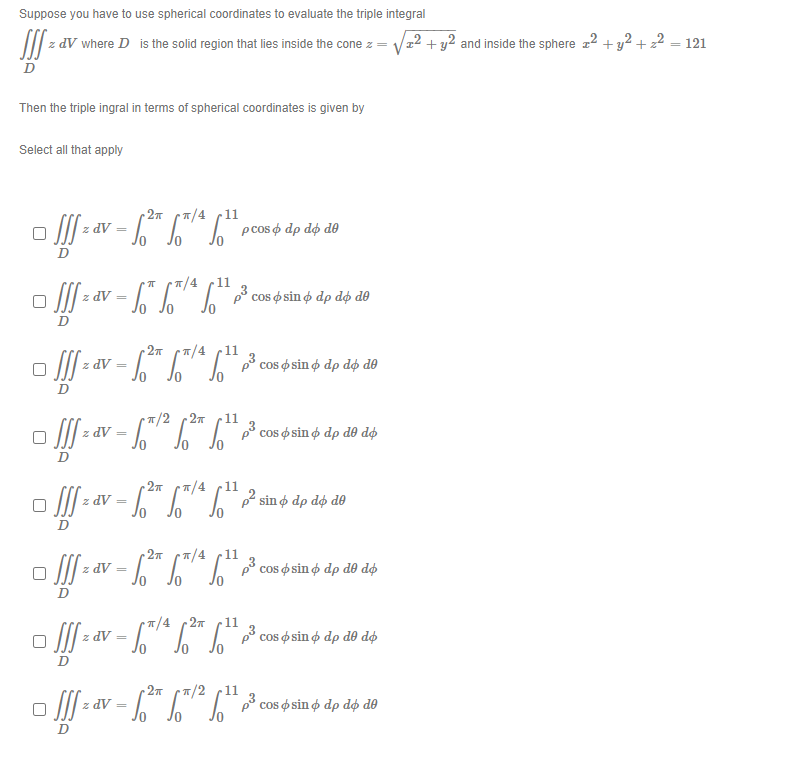Suppose you have to use spherical coordinates to evaluate the triple integral III z av where D is the solid region that lies inside the cone z = /22 + y2 and inside the sphere 22 + y2 + 2 = 121 D Then the triple ingral in terms of spherical coordinates is given by Select all that apply pcos o dp do de z dV = cos sin o dp do de D z DV = D pocos o...

• ### For the described solid S, write the triple integral f(x,y, z)dV as an iterated integral in (i) r...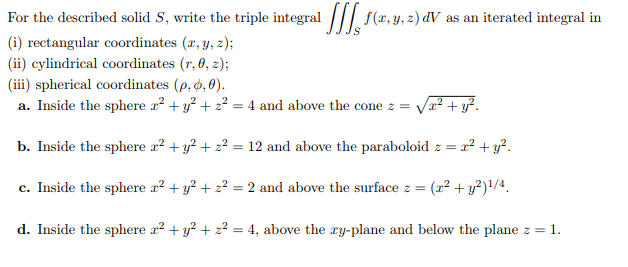For the described solid S, write the triple integral f(x,y, z)dV as an iterated integral in (i) rectangular coordinates (x,y, z); (ii) cylindrical coordinates (r, 0, 2); (iii) spherical coordinates (p, φ,0). a. Inside the sphere 2 +3+224 and above the conezV b. Inside the sphere x2 + y2 + 22-12 and above the paraboloid z 2 2 + y2. c. Inside the sphere 2,2 + y2 + z2-2 and above the surface z-(z2 + y2)1/4 d. Inside the sphere...

• ### 6) Consider the solid region E bounded by x-0, x-2, 2-y, 2-y-1, 2-0, and 24, set up a triple inte...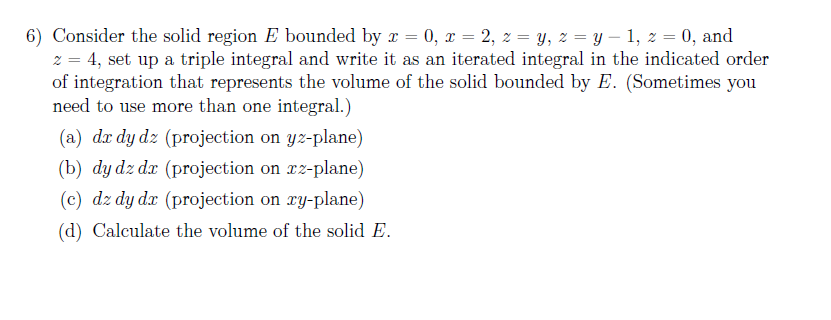6) Consider the solid region E bounded by x-0, x-2, 2-y, 2-y-1, 2-0, and 24, set up a triple integral and write it as an iterated integral in the indicated order of integration that represents the volume of the solid bounded by E. (Sometimes you need to use more than one integral.) (a) da dy dz (projecti (b) dy dz dr (projection on rz-plane) (c) dz dy dx (projection on ry-plane) (d) Calculate the volume of the solid E on...